# ISEE Lower Level Math : How to find the perimeter of a triangle

## Example Questions

← Previous 1 3 4 5 6

### Example Question #1 : How To Find The Perimeter Of A Triangle

The triangle below has a perimeter of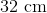. The lengths of two sides are shown below.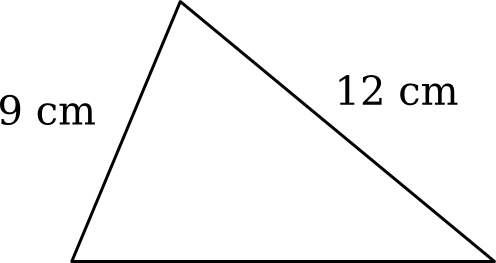What is the length of the third side?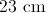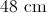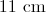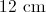Explanation:

Remember, the perimeter of a triangle is the sum of its three sides. First, sum up the two side lengths that we are given in the question: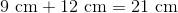To find the third side, subtract the sides we know from the total perimeter: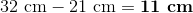### Example Question #1 : How To Find The Perimeter Of A Triangle

An equilateral triangle has a perimeter of 42 cm. WHat is the length of one of its sides?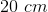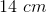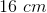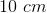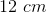Explanation:

By definition, an equilateral triangle is a triangle with three equal sides. That is, the length of each side of the triangle is going to be the same.

In this problem, we know that the perimeter (the sum of all the lengths) is 42 cm.

Side 1 + Side 2 + Side 3 = 42 cm.

Since the sides are equal, we can write the following equation.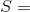one side of the triangle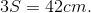To find the length of one side of the triangle, we would then divide 3 from both sides.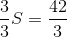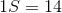Remember that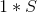is the same as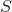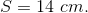### Example Question #3 : How To Find The Perimeter Of A Triangle

An equilateral triangle has a perimeter of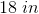.  How long is each side?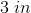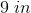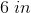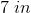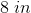Explanation:

An equilateral triangle has three congruent sides, i.e. the sides are all the same length.

The perimeter of an equilateral triangle is given by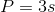.

Thus,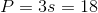; then divide each side by three to get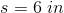.

### Example Question #1 : How To Find The Perimeter Of A Triangle

Use the triangle to answer the question.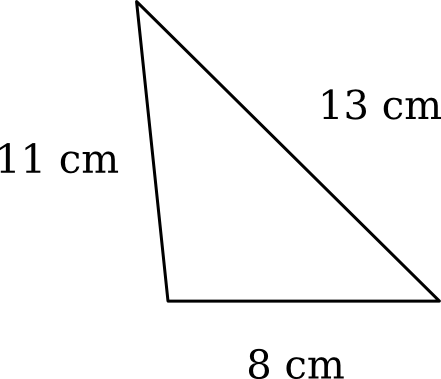What is the perimeter of the triangle?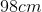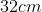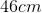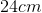Explanation:

To find the perimeter, add all the sides together.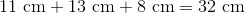### Example Question #1 : How To Find The Perimeter Of A Triangle

An equilateral triangle has a side length equal to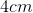.

What is the perimeter of the triangle?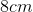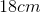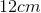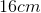Explanation:

Because it is an equilateral triangle, all sides have the same length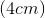.

The perimeter is the sum of the length of the sides.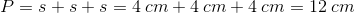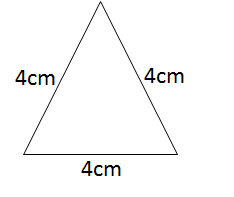### Example Question #1 : How To Find The Perimeter Of A Triangle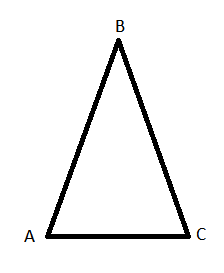Figure not drawn to scale

The perimeter of isosceles triangle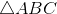is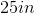.

If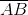and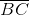are equal to each other and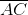is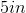, what is the length of?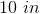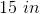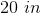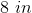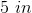Explanation:

The perimeter of the triangle is equal to the sum of the lengths of all the sides.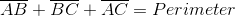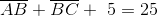Because the triangle is an isosceles triangle, the triangle has two equal sides, which we know areandSince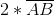is the same as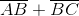, we can say: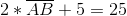.

We now solve for:Subtractfrom both sides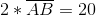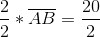Divide both sides by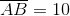### Example Question #1 : How To Find The Perimeter Of A Triangle

What is the name of the longest side of a right triangle?

Equilateral

Base

Length

Hypotenuse

Obtuse

Hypotenuse

Explanation:

The hypotenuse is the longest side of a triangle with a right angle.

All the other terms refer to something else. Obtuse refers to an angle over 90 degrees. Length is too general of a term, and refers to the measurement of any given side. Equilateral refers to a triangle in which all angles and side are of equal measurement. Base refers to the side that is perpendicular to the measure of the height, meaning that it will be one of the shorter sides.

### Example Question #8 : How To Find The Perimeter Of A Triangle

In an isosceles triangle, two side are 4 feet long. Which of the following is NOT a possible length of the third side?

2.5 feet

5 feet

1 foot

8 feet

7 feet

8 feet

Explanation:

For triangles, it is impossible for a third side to be equal to or greater than the sum of the two other sides.

Given that the sum of the two sides of this triangle is 8, it is impossible for the third side to be equal to 8 feet.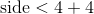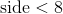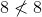### Example Question #2 : How To Find The Perimeter Of A Triangle

In an equilateral triangle, the perimeter is 42. What is the length of 1 side?Explanation:

In an equilateral triangle, all 3 sides are equal to one another. Therefore, if the perimeter is 42, the length of 1 side will be equal to 42 divided by 3. This results in 14, which is therefore the correct answer.

### Example Question #1 : How To Find The Perimeter Of A Triangle

If the side of an equilateral triangle is equal to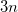and the perimeter is equal to 18, what is the value of?Explanation:

The three sides must sum to 18: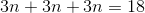Combine like terms on the left: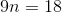Divide both sides by 9: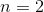← Previous 1 3 4 5 6

### All ISEE Lower Level Math Resources